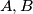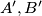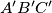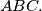### IMO Shortlist 1988 problem 3

Kvaliteta:
Avg: 0,0
Težina:
Avg: 0,0
The triangle$ABC$ is inscribed in a circle. The interior bisectors of the angles$A,B$ and$C$ meet the circle again at$A', B'$ and$C'$ respectively. Prove that the area of triangle$A'B'C'$ is greater than or equal to the area of triangle$ABC.$
Izvor: Međunarodna matematička olimpijada, shortlist 1988